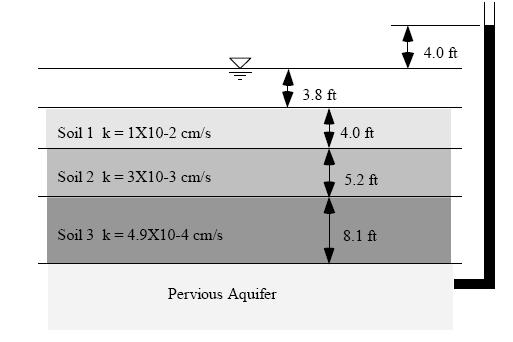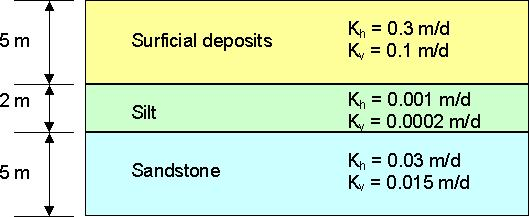# Fall Semester 2018

 Page 1 Page 2 Page 3 [Page 4] Page 5

Essay Questions

Click here to access the Equation Sheet.

22. (head-darcy) An artesian aquifer is overlain by three soil layers and a small pond as shown below. How much water flows into the pond (in ft^3) from the aquifer per square foot of area in one month's (30 days) time?23. (modflow) What is a drain? How are drains simulated in MODFLOW?

24. (head-darcy) For a soil deposit with horizontal layering of heterogeneous deposits, which is
greater, Keq for the horizontal direction or Keq for the vertical direction? Why?

25. (modflow) Enter a short description for each of the following terms associated with the Evapotranspiration package:

a. Max ET Rate
b. ET Surface
c. Extinction Depth

26. (gw-concepts) Describe the three basic types of ground water models.

27. (head-darcy) The water level in an observation well A is measured at 3785.4 ft. At a point 1238 ft downgradient from that point, the water level in a second well (B) is measured at 3629.3 ft.

a. What is the hydraulic gradient between the well A and well B?
b. Assuming that the hydraulic conductivity is 3.0 ft/day and the effective porosity is 0.3, what is the seepage velocity between the two points? [ft/day]
c. How long would it take for a particle to travel from point A to point B? [days]